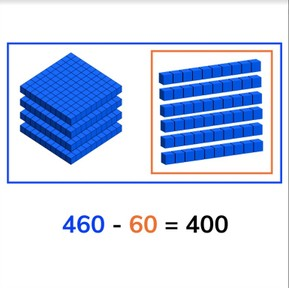Subtracting numbers to a hundreds number

# Subtracting numbers to a hundreds number8,000 schools use Gynzy92,000 teachers use Gynzy1,600,000 students use Gynzy

## General

Students learn to subtract numbers to a hundreds number.

2.NBT.B.7

## Relevance

Discuss with students that it is important to be able to subtract to a hundreds number, so you can determine how many you have left.

## Introduction

Show students the number sequence on the interactive whiteboard and ask students to count forward. A few of the numbers are already given. Then ask students to complete the number sequence counting backward using the beach balls. Next show students the MAB blocks on the interactive whiteboard. Ask students to determine how many hundreds, tens and ones there are. Fill in the missing numbers and then add them together to determine the total number of blocks.

## Development

Start by reminding students that hundreds are a group of one hundred and always end with a zero in the tens and ones places (00). Next explain to students that you can subtract by counting. Start by counting how many there are in total. That is the first number of your subtraction problem (the minuend). Then count how many are taken away. This is the second number of your subtraction problem (the subtrahend). When you take the second away from the first, you are left with the difference. Show this on the interactive whiteboard using the blocks and explain the different subtraction problems. Check that students are able to subtract the blocks. Then explain a different kind of subtraction problem. Explain to students that you first look at how many blocks there are in total, and at what the difference is. Then you need to determine how many are taken away. Then explain to students that you can subtract numbers in different ways. You can use a number line. When you need to determine the second number (the subtrahend) you can also make an alternate subtraction problem with the first number (the minuend) and the difference. Emphasize to students that you need to look carefully at the subtraction problems to determine how to solve the subtraction problem in the easiest or best way. Ask students to solve a few subtraction problems. Ask them to explain their strategy. Finally show students how to solve a story problem. They must first determine what kind of problem it is, which numbers are required for the subtraction problem, to say or write the problem, and then to solve the problem. Solve one story problem as a class and then ask students to solve a story problem in pairs or individually.

Check that students are able to subtract numbers to a hundreds number by asking the following question:
- What steps do you take to solve a story problem?
- How do you calculate the problems: 450 - 50 = ? and 684 - _ = 400?

## Guided practice

Students are asked to solve subtraction problems by making jumps on the number line as well as with visual support and in the abstract. Students are also given story problems to solve.

## Closing

Discuss with students that it is important to be able to subtract to a hundreds number, so you can easily determine how many you have left. Show subtraction problem on the interactive whiteboard and ask students to solve using the number line on their own sheet of paper. Have students discuss in pairs which jumps they took to solve the problem. As a class discuss which jumps were taken and that students can take the jumps that feel most comfortable for them. Then ask the students which number is being covered in the subtraction problem. After giving the class time to figure it out, ask one student to give their answer and to explain their thinking. Drag the cover away to check their answer. Then give a story problem and ask students which numbers are important for the math problem. Solve the problem as a class and ask students to explain the steps.

## Teaching tips

Students who have difficulty with this learning goal can make use of a number line to help support their subtraction. Remind students that they can take different jumps on the number line.

### The online teaching platform for interactive whiteboards and displays in schools

• Save time building lessons

• Manage the classroom more efficiently

• Increase student engagement